## Hypersphere

The-hypersphere (often simply called the-sphere) is a generalization of the Circle (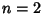) and Sphere (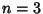) to dimensions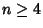. It is therefore defined as the set of-tuples of points (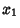,, ...,) such that(1)

whereis the Radius of the hypersphere. The Content (i.e.,-D Volume) of an-hypersphere of Radiusis given by(2)

where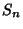is the hyper-Surface Area of an-sphere of unit radius. But, for a unit hypersphere, it must be true that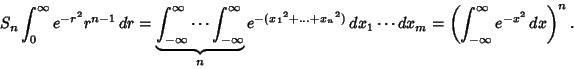(3)

But the Gamma Function can be defined by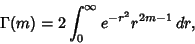(4)

so(5)(6)

This gives the Recurrence Relation(7)

Using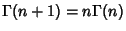then gives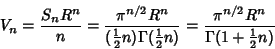(8)

(Conway and Sloane 1993).Strangely enough, the hyper-Surface Area and Content reach Maxima and then decrease towards 0 asincreases. The point of Maximal hyper-Surface Area satisfies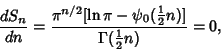(9)

where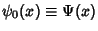is the Digamma Function. The point of Maximal Content satisfies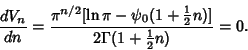(10)

Neither can be solved analytically for, but the numerical solutions arefor hyper-Surface Area andfor Content. As a result, the 7-D and 5-D hyperspheres have Maximal hyper-Surface Area and Content, respectively (Le Lionnais 1983).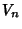0 1 1 0 1 2 1 2 2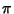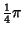345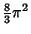6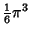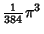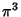7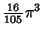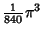8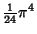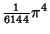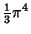9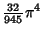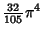10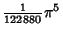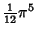In 4-D, the generalization of Spherical Coordinates is defined by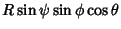(11)(12)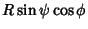(13)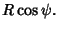(14)

The equation for a 4-sphere is(15)

and the Line Element is(16)

By defining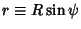, the Line Element can be rewritten(17)

The hyper-Surface Area is therefore given by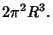(18)

References

Conway, J. H. and Sloane, N. J. A. Sphere Packings, Lattices, and Groups, 2nd ed. New York: Springer-Verlag, p. 9, 1993.

Le Lionnais, F. Les nombres remarquables. Paris: Hermann, p. 58, 1983.

Peterson, I. The Mathematical Tourist: Snapshots of Modern Mathematics. New York: W. H. Freeman, pp. 96-101, 1988.